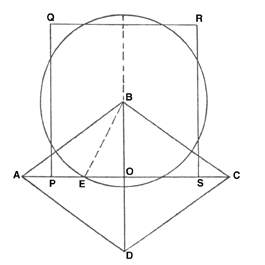# Geometry of the Ideal Bee’s Cell

Geometry of the Ideal Bee’s Cell

# Geometry of the Ideal Bee’s Cell

The Bee World – June, 1944 – Page 46

MISCELLANY

Geometry of the Ideal Bee’s Cell. The bee’s cell was the object of much discussion by amateur mathematicians (and some who were more eminent) in the 18th and 19th centuries. A good deal of nonsense was written on the subject, for many of the writers did not realise how seldom bees build cells conforming to the ideal pattern, nor that the resulting cell-form depends much more upon the stresses in the semi-plastic wax inside the warm building-cluster than upon such considerations as an instinct for using the least amount of wax possible. See B.W. 3, 37, for the history of the problem.None of the investigators seem, however, to have hit upon the interesting approximate solution of the problem of “squaring the circle” provided by the so-seldom-realised ideal cell. ABCD is one of the rhombs or lozenges from the base of such a cell, AC and BD its diagonals, meeting at O. Bisect the angle ABO by line BE. With BE as radius and centre B, describe a circle, cutting AC at E. The square on BD (or 2 x BO) is nearly equal to the area of this circle. The proof is easy, recalling that BO, OA and AB are in the proportions of the square roots of 1, 2 and 3 to one another; while OB/BA, being the cosine of the angle ABO (or 2 x angle EBO) is, by a well-known rule, equal to 2(OB/EB)2-1.

Now (2.OB/BE)2=(area of square PQRS divided by square of radius BE)=”pi,” nearly. This, from the above, is = 2(OB/BA+1) = 2(1/3 of the square root of 3+1)=2×1.57735=3.1547.

This is not a very close approximation, to “pi,” being about 4% out. But it is closer than the Egyptians’ value, the square of 16/9, or 3.1605. The accurate value, to 4 places of decimals, is 3.1416; and the usual rough approximation 22/7, is about 0.4% out.

A.D.B.